##### Organic Chemistry I Workbook For Dummies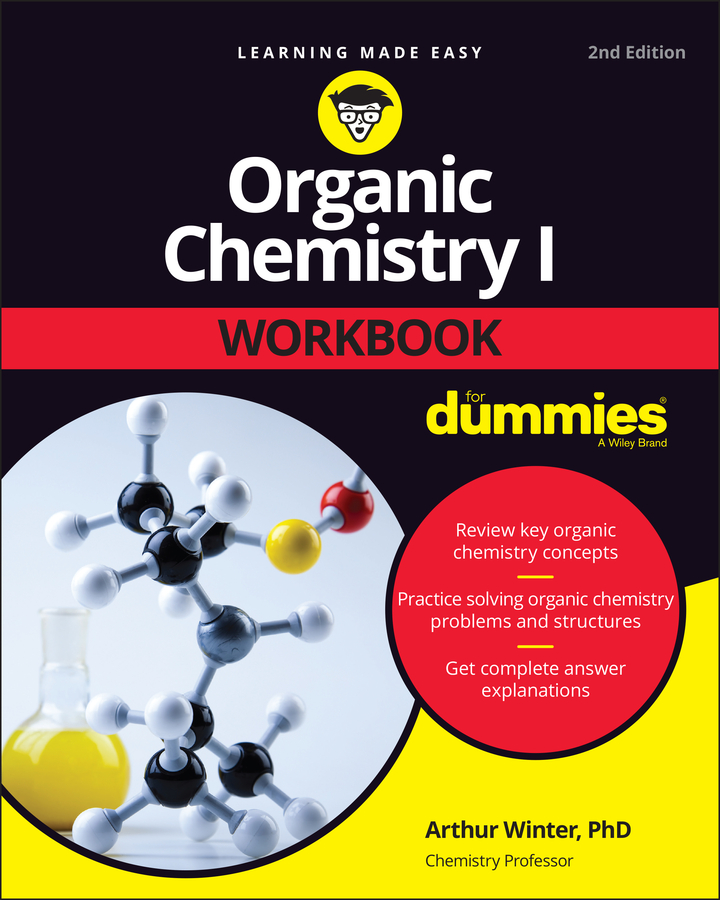Two important ways to measure concentration are molarity and percent solution. Different solutes dissolve to different extents in different solvents in different conditions. To keep track of all these differences, chemists measure concentration. Qualitatively, a solution with a large amount of solute is said to be concentrated. A solution with only a small amount of solute is said to be dilute. Quantitatively, we use numbers; hence, molarity and percent solution.

Molarity relates the amount of solute to the volume of the solution: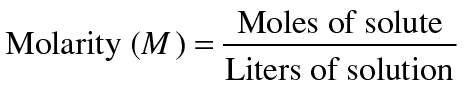To calculate molarity, you may have to use conversion factors to move between units. For example, if you're given the mass of a solute in grams, use the molar mass (usually rounded to two decimal places) of that solute to convert the given mass into moles. If you're given the volume of solution in milliliters or some other unit, you need to convert that volume into liters. Units are the very first thing to check if you get a problem wrong when using molarity. Make sure your units are correct!

The units of molarity are always moles per liter (mol/L or mol·L1). These units are often abbreviated as M and referred to as "molar." Thus, 0.25 M KOH(aq) is described as "Point two-five molar potassium hydroxide," and it contains 0.25 mol of KOH per liter of solution. Note that this does not mean that there are 0.25 mol KOH per liter of solvent (water, in this case) — only the final volume of the solution (solute plus solvent) is important in molarity.

Like other units, the unit of molarity can be modified by standard prefixes, as in millimolar (mM, which equals 103 mol/L) and micromolar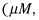which equals 106 mol/L).

Percent solution is another common way to express concentration. The precise units of percent solution typically depend on the phase of each component. For solids dissolved in liquids, mass percent is usually used: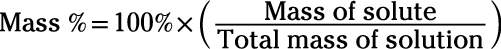Sometimes, the term percent solution is used to describe concentration in terms of the final volume of solution instead of the final mass. For example:

• "5% Mg(OH)2" can mean 5 g magnesium hydroxide in 100 mL final volume. This is a mass-volume percent solution.

• "2% H2O2" can mean 2 mL hydrogen peroxide in 100 mL final volume. This is a volume-volume percent solution.

Clearly, paying attention to units is important when working with concentration. Only by observing which units are attached to a measurement can you determine whether you're working with molarity, with mass percent, or with a mass-mass, mass-volume, or volume-volume percent solution.

Here's an example: Calculate the molarity and the mass-volume percent solution obtained by dissolving 102.9 g H3PO4 into 642 mL final volume of solution. Be sure to use proper units. (Hint: 642 mL = 0.642 L)

First, calculate the molarity. Before you can use the molarity formula, though, you must convert grams of H3PO4 to moles: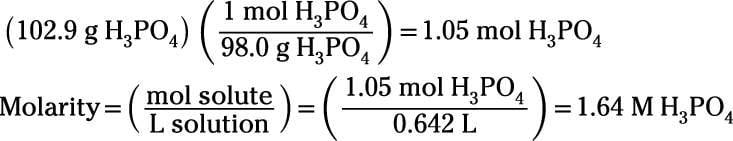Next, calculate the mass-volume percent solution: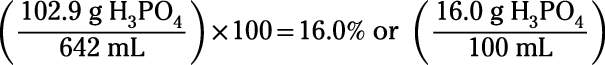Note that the convention in molarity is to divide moles by liters, but the convention in mass percent is to divide grams by milliliters. If you prefer to think only in terms of liters (not milliliters), then simply consider mass percent as kilograms divided by liters.